# Difference between revisions of "Stokes-Einstein relation"This article is a 'stub' page, it has no, or next to no, content. It is here at the moment to help form part of the structure of SklogWiki. If you add sufficient material to this article then please remove the {{Stub-general}} template from this page.

The Stokes-Einstein relation, originally derived by William Sutherland (Ref. 1) but almost simultaneously published by Einstein (Ref. 2), states that, for a sphere of radius$R$ immersed in a fluid,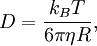$D=\frac{k_B T}{6\pi\eta R},$

where D is the diffusion constant,$k_B$ is the Boltzmann constant, T is the temperature and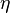$\eta$ is the viscosity. Sometimes, the name is given to the general relation: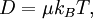$D=\mu k_B T,$

where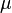$\mu$ is the mobility. This, coupled with Stokes' law for the drag upon a sphere moving though a fluid: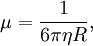$\mu=\frac{1}{6\pi\eta R} ,$

produces the first equation.